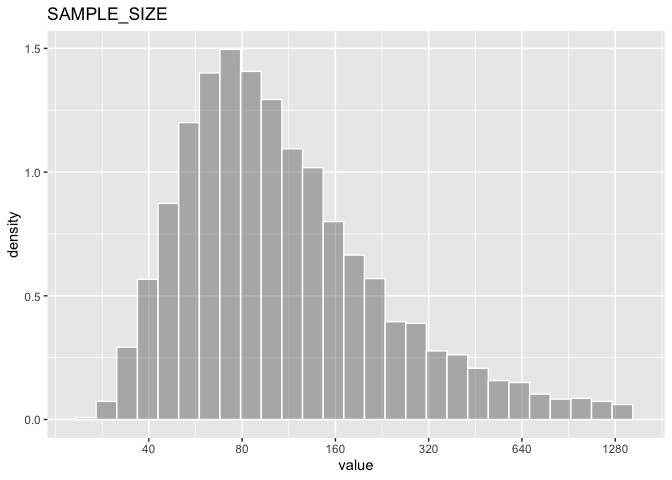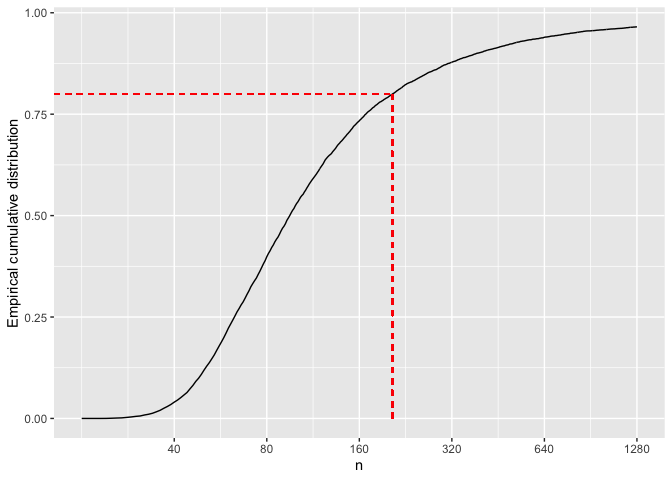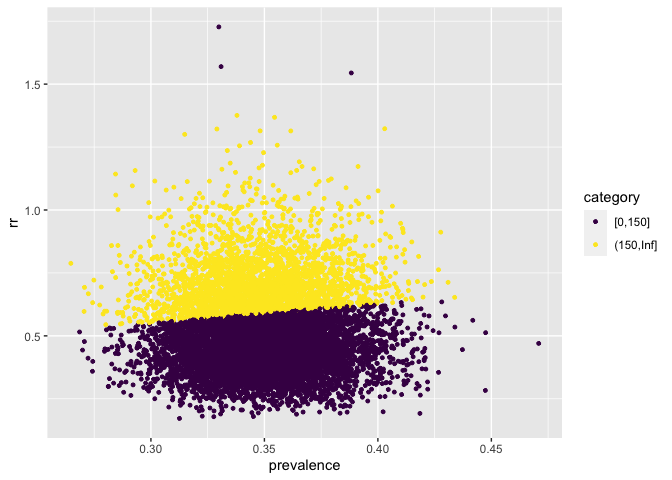# Sample size with Uncertainty

## Introduction

This example show in details how to construct a new factory of DISTRIBUTION objects using a convolution of parameters to propagate the uncertainty to the final calculation.

The estimation of sample size require the input of different parameters in a way that when the parameters are correct, the power to have a significant result is correct. However many times there are some uncertainty on the parameters and this is not reflected in the power.

The convdist package could help on explicitly express uncertainty on those parameters and estimate the distribution of sample sizes that would satisfy the power requirements of the assay.

We explore how to estimate the sample size for binomial outcome with uncertainty and explain the details of how to construct a DISTRIBUTION factory function.

## Sample size for a proportion

For an individually randomized trial, the number of subjects per group could be defined as $n = (z_{\alpha/2} + z_\beta)^2 [\pi_0(1 – \pi_0) + \pi_1(1 – \pi1)]/(\pi_0 – \pi_1)^2$

In this formula, $$z_{\alpha/2}$$ and $$z_\beta$$ are standard normal distribution values corresponding to upper tail probabilities of $$z_{\alpha/2}$$ and $$z_\beta$$ respectively. This choice of sample size provides a power of 100(1 – $$\beta$$)% to obtaining a significant difference ($$P < \alpha$$ on a two-sided test), assuming that the true (population) proportion in presence or absence of the interventions are $$\pi_1$$ and $$\pi_0$$ respectively

$$\pi_1$$ can be express on terms of $$\pi_0$$ as $$\pi_1 = \pi_0 RR$$ where $$RR$$ is the relative risk.

In the real world, there could be uncertainty on the exact values of $$\pi_0$$ and $$RR$$ as they may come from previous studies or surveys. What would be the distribution of $$n$$ if the uncertainty is taking into account?

## A new DISTRIBUTION factory

We can construct a DISTRIBUTION object which would produce random numbers taking into account the a probability distribution for $$\pi_0$$ and $$RR$$

See the code of new_UNIFORM function as an example of how is a factory of DISTRIBUTION objects:

library(convdistr)
#> Registered S3 method overwritten by 'pryr':
#>   method      from
#>   print.bytes Rcpp
new_UNIFORM
#> function (p_min, p_max, p_dimnames = "rvar")
#> {
#>     stopifnot(p_min <= p_max)
#>     .oval = (p_max + p_min)/2
#>     names(.oval) <- p_dimnames
#>     structure(list(distribution = "UNIFORM", seed = sample(1:2^15,
#>         1), oval = .oval, rfunc = restrict_environment(function(n) {
#>         matrix(runif(n, p_min, p_max), ncol = 1, dimnames = list(1:n,
#>             p_dimnames))
#>     }, p_min = p_min, p_max = p_max, p_dimnames = p_dimnames)),
#>         class = c("UNIFORM", "DISTRIBUTION"))
#> }
#> <bytecode: 0x7f8c15181318>
#> <environment: namespace:convdistr>

The function should return an structure which consist of a list of four named vector and a class attribute that should include “DISTRIBUTION” The components of the list are:

distribution : A character with the name of the distribution implemented. It is a good practice to use the same name also in the class attribute

seed : A numerical seed that is use to get a repeatable sample in the summary function Use a sample function to ensure each new object will have a different seed

oval : The observed value. It is the value expected. It is used as a number for the mathematical operations of the distributions as if they were a simple scalar

rfunc(n) : A function that generate random numbers from the distribution. Its only parameter n is the number of drawns of the distribution. It returns a matrix with as many rows as n, and as many columns as the dimensions of the distributions, The rfunc should be defined within a restrict_environment function to ensure only the variables required to the function are included in the environment of the function. This will prevent reference leaking which could make the objects big and slow when saved in a file.

The factory of object for the sample size of proportions could be defined as:


#' Sample size for proportions, with uncertainty
#'
#' Create an new DISTRIBUTION object that produce
#' random drawns of the estimated sample size for
#' two proportions
#' @param p0 a DISTRIBUTION object that drawns for proportions in control group
#' @param logrr a DISTRIBUTION object that drawns log(RR) of the intervention
#' @param alpha significant value
#' @param beta  1-power
new_SAMPLE_SIZE <- function(p0,logrr, alpha= 0.05, beta=0.2){
#checkings
stopifnot(inherits(p0,"DISTRIBUTION"))
stopifnot(inherits(logrr,"DISTRIBUTION"))
stopifnot(0 < alpha & alpha < 1)
stopifnot(0 < beta & beta < 1)

# function of alpha and beta
f_alpha_beta <- (qnorm(alpha/2,lower.tail = F) + qnorm(beta, lower.tail = F)) ^ 2

# The expected value of the distribution with default name for the dimension
# based on the oval value of the individual distributions
v0 <- p0$oval v1 <- p0$oval*exp(logrr$oval) # expected value of the distribution .oval <- f_alpha_beta * (v0 * (1 - v0) + v1 * (1 - v1)) / (v0 - v1) ^ 2 names(.oval) <- "rvar" #random function within a restricted environment where only #the specified variables can be accesed within the function .rfunc <- restrict_environment( function(n) { d_p0 = rfunc_p0(n) d_p1 = d_p0 * exp(rfunc_logrr(n)) fab * (d_p0 * (1 - d_p0) + d_p1 * (1 - d_p1)) / (d_p0 - d_p1) ^ 2 }, rfunc_p0 = p0$rfunc,
rfunc_logrr = logrr$rfunc, fab = f_alpha_beta ) # Create the object with 4 slots structure( list( distribution = "SAMPLE_SIZE", seed = sample(1:2 ^ 15, 1), oval = .oval, rfunc = .rfunc ), class = c("SAMPLE_SIZE","DISTRIBUTION") ) } ## Using the Sample Size object As example, suppose that the incidence of anaemia in children under 5 have been estimated from a survey as 35% with 95%CI (30%,40%), and a prevention treatment that in other settings have shown to decrease anaemia by 50% with 95%CI(8% to 73%). We can define a BETA distribution based on the CI as d_p0 = new_BETA_lci(0.35,0.30,0.40) And a NORMAL distribution of the log(RR) as rr = log(1 - 50/100) sd = (log((1 - 8 / 100)) - log((1 - 73 / 100))) / 4 d_logrr = new_NORMAL(rr,sd) So the input distributions are distribution varname oval nsample mean_ sd_ lci_ median_ uci_ BETA rvar 0.35 10000 0.35 0.02 0.30 0.35 0.40 NORMAL rvar -0.69 10000 -0.70 0.31 -1.31 -0.70 -0.09 And base on this we can calculate the distribution of samples with those conditions d_sample <- new_SAMPLE_SIZE(d_p0, d_logrr) distribution varname oval nsample mean_ sd_ lci_ median_ uci_ SAMPLE_SIZE rvar 95 10000 47208 3172752 37 97 2446The oval value of the distribution give us the result we obtain from the formula. With the conditions specified, a sample size of 95 is required. However, the distribution is quite skewed as you can see the mean and the median value are far, and the standard deviation very big. This is because the sample size require for combinations of low efficacy and low prevalence could be very large and there is no restriction on how low could be the efficacy or the prevalence, which although have low frequency, could happen in the simulations. rdrawn <- rfunc(d_sample, 10000) f_ecdf <- ecdf(rdrawn) n_80 = trunc(uniroot(function(x){f_ecdf(x) - 0.8}, interval = c(10,10000))$root)

The empirical cumulative distribution values of a random sample of the distribution show us that with 205 subjects per arm, 80% of the possible scenarios are covered## Enhance the Sample Size object

The function created above show us a distribution of sample size, but we can’t inspect condition on which a particular drawn is obtained, i.e. how low an prevalent anaemia value could be compensated with a high efficacy value? To do that we could create a second object that have drawns in several dimensions, the prevalence, the RR and the sample size so we can make a graph with the three values.

#' Sample size for proportions, with uncertainty (V2)
#'
#' Create an new DISTRIBUTION object that produce
#' random drawns of the estimated sample size for
#' two proportions as well as the samples from the parameters
#' @param p0 a DISTRIBUTION object that drawns for proportions in control group
#' @param logrr a DISTRIBUTION object that drawns log(RR) of the intervention
#' @param alpha significant value
#' @param beta  1-power
new_SAMPLE_SIZE2 <- function(p0,logrr, alpha= 0.05, beta=0.2){
#checkings
stopifnot(inherits(p0,"DISTRIBUTION"))
stopifnot(inherits(logrr,"DISTRIBUTION"))
stopifnot(0 < alpha & alpha < 1)
stopifnot(0 < beta & beta < 1)

# function of alpha and beta
f_alpha_beta <- (qnorm(alpha/2,lower.tail = F) + qnorm(beta, lower.tail = F)) ^ 2

# The expected value of the distribution with default name for the dimension
# based on the oval value of the individual distributions
v0 <- p0$oval v1 <- p0$oval*exp(logrr$oval) # expected value of the distribution ss <- f_alpha_beta * (v0 * (1 - v0) + v1 * (1 - v1)) / (v0 - v1) ^ 2 .oval = c(p0$oval, exp(logrr$oval), ss) names(.oval) <- c('prevalence', 'rr', 'sample_size') #random function within a restricted environment where only #the specified variables can be accesed within the function .rfunc <- restrict_environment( function(n) { d_p0 = rfunc_p0(n) d_rr = exp(rfunc_logrr(n)) d_p1 = d_p0 * d_rr d_ss = fab * (d_p0 * (1 - d_p0) + d_p1 * (1 - d_p1)) / (d_p0 - d_p1) ^ 2 matrix( c(d_p0, d_rr, d_ss), ncol = 3, dimnames = list(1:n, c('prevalence','rr','sample_size'))) }, rfunc_p0 = p0$rfunc,
rfunc_logrr = logrr$rfunc, fab = f_alpha_beta ) # Create the object with 4 slots structure( list( distribution = "SAMPLE_SIZE2", seed = sample(1:2 ^ 15, 1), oval = .oval, rfunc = .rfunc ), class = c("SAMPLE_SIZE2","DISTRIBUTION") ) } d_sample2 <- new_SAMPLE_SIZE2(d_p0, d_logrr) distribution varname oval nsample mean_ sd_ lci_ median_ uci_ SAMPLE_SIZE2 prevalence 0.35 10000 0.35 0.02 0.30 0.35 0.40 SAMPLE_SIZE2 rr 0.50 10000 0.52 0.16 0.28 0.50 0.92 SAMPLE_SIZE2 sample_size 95.31 10000 1286105.81 89687564.95 38.00 95.31 2677.42 ss <- rfunc(d_sample2, 10000) df <- data.frame(ss) df$category = cut(df\$sample_size, c(0,150,Inf),include.lowest = T, ordered_result = T)
#> 6  0.3332922 0.6896455   292.86259 (150,Inf]